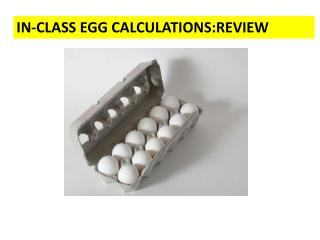Download PresentationIN-CLASS EGG CALCULATIONS:REVIEWIN-CLASS EGG CALCULATIONS:REVIEW - PowerPoint PPT Presentation

Download PresentationIN-CLASS EGG CALCULATIONS:REVIEW
An Image/Link below is provided (as is) to download presentation

Download Policy: Content on the Website is provided to you AS IS for your information and personal use and may not be sold / licensed / shared on other websites without getting consent from its author. While downloading, if for some reason you are not able to download a presentation, the publisher may have deleted the file from their server.

- - - - - - - - - - - - - - - - - - - - - - - - - - - E N D - - - - - - - - - - - - - - - - - - - - - - - - - - -
Presentation Transcript

1. IN-CLASS EGG CALCULATIONS:REVIEW

2. IN-CLASS simple mole calculations (on board) the dozen method way

3. EGG WORLD CHEMISTRY WORLD Smallest unit 1 egg 1 molecule Practical Counting unit Mole (The chemist’s dozen) Dozen

4. Eggs vs. chemistry world (continued) EGG WORLD CHEMISTRY WORLD # of smallest units in practical counting unit 1 mole  6.02217 *1023 1 dozen=12 (Avogadro’s #) grams/mole =gram molecular weight = MW (molecular weight) (ADD) Practical mass unit grams/dozen (WEIGH)

5. THE ONE PLACE EGG WORLD AND CHEMISTRY WORLD DEVIATE A TEENY BIT: Egg world Chemistry world Mass dozen Mass = molecular weight mol (MW) Just add up atomic masses using Periodic Table in grams Must weigh box of eggs

6. Mass (grams)= MW=Molecular mol Weight = sum of masses in grams of component elements in a compound . (element masses are average masses taken from Periodic Table)

7. Example of MW Calculation: What is theMWof methane =CH4 ?? atomic mass (Table) # atoms/molecule product C H 1 4 ~12*1=12 ~1*4 = 4 12.011~12 1.00794~1 Sum = MW = 12+4=16 g/mole

8. In – class exercise on Molecular Weight (MW) calculations Find the MW of… H2SO4 CaCl2 Cu3(PO4)2 2*1 + 1*32 + 4*16 = 98 g/mol 1*40 + 2*35.4 = 110.4 g/mol 3*63.5 + 2*(1*31 +4*16) = 380.5 g/mol 2 `waters of hydration’ per CaCl2 CaCl2*2H2O 1*40 + 2*35.4 + 2*(2*1 + 1*16)=146.8 g/mol

9. Another way to view mole calculations (if you are allergic to eggs): the triangleMap Method Moles (n) Weight (w)molecule count (N) ALL ROADS LEAD THROUGH MOLES

10. The triangle `trick’ Multiply Down Moles (n) Weight (w)molecule count (N) Divide up

11. mole math the triangle way You always get MWand Avogadro’s # = 6.02*1023 Moles (n) w= n x MW N= n*6.02*1023 If you have moles they multiply !! (because… moles breed whenever they can) Molecule count (N) Weight (w)

12. reverse mole math the triangle way Moles (n) n= N 6.02*1023 n = w MW Ifyou want moles …don’t let these horny critters multiply…divide them up!!! Weight (w) Molecule count (N)

13. Trips around the triangle: Nw Given: 100 g/mol =MW Given: chemist’s dozen =1 mol count=6.02*1023 Moles (n) w=n*MW =20* 100 6.02*1023 n= 20 6.02*1023 All roads lead through moles Given molecule count N=20 Weight (w) ? w~3.3*10-21 g

14. Trips around the triangle: wN Given : chemist’s dozen =1 mol count=6.02*1023 Given: 100 g/mol =MW N=n x 6.02*1023 =200 x 6.02*1023 100 n= w = 200 MW 100 Moles (n) All roads lead through moles Given weight w=200 g Molecule count N ? N=1.2*1024

15. AN ANECDOTE An `out-of-towner’ visiting NYC for the first time asks Joshua Bell (world’s greatest violinist): “How do you get to the Lincoln Center for the Performing Arts ?” Joshua’s answer (supposedly): “Practice, practice, practice….”

16. Exercise #3a: mole calculations part 1 • weight per mole (MW): what does a mole • of C6H12O6weigh ? (C=12; H=1; O=16) 6*12 + 12*1 + 6*16 = 180 g/mol 1.1) mole to mass: how many g C6 H12O6 of are present in 0.00555 mole of C6 H12O6? 0.00555 mol * 180 g/mol = 1 g 1.2) mass to mole: how many moles of C6 H12O6 are in 360 g of C6H12O6? 360 g/180 g mol-1 = 2 moles

17. Exercise #3a: mole calculations part 1 (continued) 1.3) mole to # molecule: how many molecules of C6 H12O6 are in 0.5 mol of C6H12O6 ? 0.5 mol *6*1023 molecules/mol = 3*1023molecules 1.4) mass to # molecules:how many molecules of C6 H12O6 are in 120 g of C6H12O6? (120/180) mol * 6*1023 molecules =4*1023 molecules mol

18. Exercise 5 part 1 (continued) 1.5) # molecules to moles: how many moles in 3*1024 molecules of C6 H12O6 ? 3*1024 molecules/ 6*1023 molecules/mol = 5 moles 1.6) # molecules to mass: How many grams of C6 H12O6are in 2.0 *1022 molecules of C6 H12O6 ? 2*1022 molecules * 180 g 6*1023 molecules/mol mol =0.0333 * 180 = 6 grams

19. The gram molecular mass of D form N-methylphenyl-2-propan-2-amine (the active form of ‘crystal meth’) is149.2 g/mol. How many moles of crystal meth are in a typical street `teenth’ = 1/16 of an ounce= 1.80 grams ? (Note: 1 mol count=6.022*1023.) • 7.27*1021 • 0.0121 • 82.9 • 2.48*10-22 • 8.98*1025 • 268.5 • My answer isn’t above

20. B1-Aflatoxin (MW=328.2 g/mol) is a naturally occurring, horribly toxic poison derived from Aspergillus fungi, a common contaminant in corn. It causes cancer in most humans at the staggeringly low exposure level of 1*1017 molecules. What is the equivalent mass of aflatoxin represented by this count ? (Note: 1 mol count=6.022*1023.) • 3.28*10-15 g • 1.84*10-4 g • 5.45*10-5 g • 5.06*10-10 g • 1.98*10-10 g • 6.02*106 g • My answer isn’t above

21. Micro chip manufacture requires nearly oxygen-free conditions wherein the concentration of O2is at or below 17 pg/L. Given that the atomic mass of O is16g/mol, about how many molecules of O2 /L does this represent ? Note: 1 mol count=6.022*1023 • 5.4*1024 • 3.5*1022 • 5.3*1022 • 3.2*1011 • 6.4*1012 • 1.9*1013 • My answer isn’t above